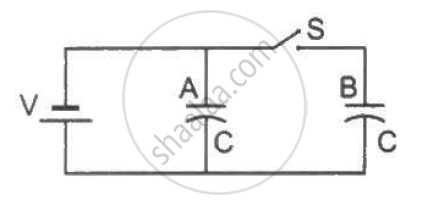Department of Pre-University Education, KarnatakaPUC Karnataka Science Class 12
Share

# Figure Shows Two Identical Parallel Plate Capacitors Connected to a Battery Through a Switch S. Initially, the Switch is Closed So that the Capacitors Are Completely Charged. - Physics

ConceptEnergy Stored in a Capacitor

#### Question

Figure shows two identical parallel plate capacitors connected to a battery through a switch S. Initially, the switch is closed so that the capacitors are completely charged. The switch is now opened and the free space between the plates of the capacitors is filled with a dielectric of dielectric constant 3. Find the ratio of the initial total energy stored in the capacitors to the final total energy stored.#### Solution

When the switch is closed, both capacitors are in parallel.

⇒ The total energy of the capacitor when the switch is closed is given by E_i = 1/2 CV^2 + 1/2 CV^2 = CV^2

When the switch is opened and the dielectric is induced, the capacitance of the capacitor A becomes

C^' = KC = 3C

The energy stored in the capacitor A is given by

E_A = 1/2 C^'V^2

⇒ E_A = 1/2 (3C)V^2 = 3/2 CV^2

The energy in the capacitor B is given by

E_B = 1/2 xx C/3 xx V^2

therefore Total final Energy

E_f = E_A + E_B

⇒ E_f = 3/2 CV^2 + 1/6 CV^2

⇒ E_f = (9 CV^2 + 1CV^2)/6 = 10/6 CV^2

Now,

Ratio of the energies, E_1/E_2 = (CV^2)/(10/6 CV^2) = 3/5

Is there an error in this question or solution?

#### Video TutorialsVIEW ALL 

Solution Figure Shows Two Identical Parallel Plate Capacitors Connected to a Battery Through a Switch S. Initially, the Switch is Closed So that the Capacitors Are Completely Charged. Concept: Energy Stored in a Capacitor.
S